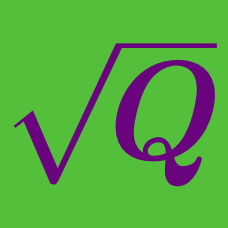Algebra

# Radical Expressions and Equations: Level 2 Challenges

$\large \sqrt { -4 } \times \sqrt { -9 } = \, ?$

$\large \dfrac{\sqrt{50}+7}{\sqrt{50}-7}+\dfrac{\sqrt{50}-7}{\sqrt{50}+7} = \ ?$

$\Huge \sqrt{\color{red}9^{\color{green}{16} \color{blue}{x^2}}}$

Which of the following is equal to the above expression?

$\Large{ \sqrt{3+2\sqrt2} - \sqrt{3-2\sqrt2} = \ ?}$

Which is greater?

$\Large\sqrt{ \color{red}{8}} - \sqrt{ \color{red}{7}} \ \ \ \ \ \text{OR} \ \ \ \ \ \sqrt{ \color{blue}{6}} - \sqrt{ \color{blue}{5}}$

×